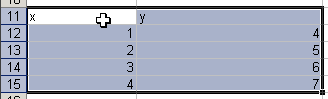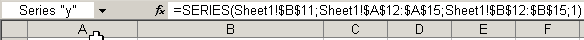# Charting a mathematical equation using Excel and defined names

## Create the Chart

Because we cannot start creating a chart without data, simply add some data to a couple of cells:and click the chart wizard button. Make sure to select the "XY (scatter)" chart type and hit Finish.

Now define two names local to the worksheet the chart is on:

Name
Refers To
Sheet1!x
\$A\$12:\$A\$15
Sheet1!y
\$B\$12:\$B\$15

Now we need to use these two defined names in the chart's SERIES formula (click the line in the chart and then click the formula bar), currently looking like this:(Note this shows the SERIES formula as I see it, with the semicolon as list separator.)

Edit this formula so it looks like this:

=SERIES(Sheet1!\$B\$8,Sheet1!x,Sheet1!y,1)

Cell B8 will contain the chart's title, so we'll put this little formula in B8:

="Charting: " & Sheet1!\$B\$1